## Thursday, December 29, 2011

### New function for comparative analysis with intraspecific data

I recently added a function to phytools called fitBayes for fitting evolutionary models with intraspecific variability. It is in version 0.1-5 (and higher) of the phytools package, and I also just posted the code to my R phylogenetics page. Note that multiple functions are required to run this analysis so it is probably better to just download the phytools package (latest nonstatic version here).

The function takes as input a species phylogeny and a vector of data for one or multiple individuals per species. It then simultaneously samples species means and variances, as well as the parameters of the fitted evolutionary model (presently BM and the λ model). The neat thing about this method is that the tree and evolutionary model can influence the estimated species means (as well as the reverse). In theory (and in simulations) this leads to more accurate estimates of the means for species than when the phylogeny is ignored.

I'm not going to go into great detail about this function - I have a submitted manuscript in which Graham Reynolds (presently a postdoc in my lab) and I describe the approach - however I have posted a little demo, with explanation, below.

First, load phytools and simulate a tree and data for the species means at the tips:

require(phytools)
set.seed(1)
tree<-pbtree(n=30,scale=1)
xbar<-fastBM(tree,sig2=0.5) # true species means

Now, we can generate a vector in which the values are sampled from a normal distribution with means given by xbar:

x<-vector()
for(i in 1:30){
n<-floor(runif(n=1,min=1,max=4))
y<-rnorm(n=n,mean=xbar[i],sd=sqrt(0.2))
names(y)<-rep(names(xbar)[i],length(y))
x<-c(x,y)
}

Now, let's run the MCMC:

X<-fitBayes(tree,x,ngen=100000,model="BM",method="reduced")

Ok, now, let's get our estimates, excluding the first 20,000 generations (201 samples) as burnin:

> results<-colMeans(X[201:1001,])
> results
gen          sig2             a       t1  ...
6.000000e+04  9.427381e-01  5.132597e-02  5.04303  ...

We can, say, plot these estimates against their generating values:

plot(xbar,results[names(xbar)],ylab="estimated means")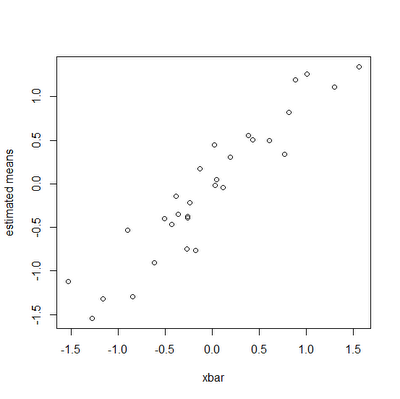We can also compare the Bayesian and standard arithmetic means quantitatively. Here, I will compute the sum of squared differences between the estimated means and the underlying generating values:

> SS.bayes<-sum((results[names(xbar)]-xbar)^2)
> SS.bayes
 2.143579
> temp<-aggregate(as.data.frame(x),list(as.factor(names(x))),mean)
> x.arith<-temp[,2]; names(x.arith)<-temp[,1]
> SS.arith<-sum((x.arith[names(xbar)]-xbar)^2)
> SS.arith
 3.953547

Here, at least, taking the tree and evolutionary model into account means we also get much better estimates of the species means. Neat.

## Wednesday, December 28, 2011

### Citing phytools

phytools is quite a new package - I posted the first release of phytools in June, and it has been available through CRAN only since late August. Several phytools functions have been cited already, but the package as a whole has not yet had time to accumulate significant citations (although a few are beginning to trickle in).

I was pleased to find today that the phytools package had been cited in a recent Journal of Experimental Biology article by Grim & colleagues. Unfortunately I was somewhat dismayed to discover that phytools was cited improperly, as follows (p. 3756):

None of the datasets, including enzyme activity, protein level and UI, included a phylogenetic signal [as indicated by Blomberg’s K using the phylogeny published by Takezaki et al. (Takezaki et al., 2003) and the ‘phytools’ package in R (R Development Core Team, 2008); P>0.05].

(In other words, phytools has been attributed to the R Development Core Team, and not to me.)

It is generally very easy to find citation information for any R package that you are using - just type citation("package-name") at the command prompt. So, for phytools this would look something like this:

> citation("phytools")

To cite phytools in publication use:

Revell, L. J. (In press) phytools: An R package for phylogenetic
comparative biology (and other things)
Methods Ecol. Evol. doi:10.1111/j.2041-210X.2011.00169.x

A BibTeX entry for LaTeX users is
...

As phytools is continually evolving, you may want to cite its version number. Find it with 'help(package=phytools)'.

Don't forget, of course, to also cite package dependencies - particularly those on which the functions that you used for your analysis rely; and the R statistical computing environment (as Grim et al. rightly do, above).

### New version of phylosig, and new functions to match data & tree

In response to a user bug report, I have now just posted a new version of the function phylosig() online. Direct link to code is here, and this will be added to the next version of phytools.

The user reported two issues:

1) If the names attribute was not assigned for the vector of standard errors, se, the method returned an error. This was not true for the data vector, x, in which phylosig just assumed the same order as tree\$tip.label for is.null(names(x))==TRUE.

2) The user also reported that the function failed for trees containing polytomies, but only in the case in which standard errors for the the trait means are provided.

First, 2). I realized quickly that this was because the function had been using pic() to get a starting value for the evolutionary rate, σ2 during optimization. This was easy enough to solve just by changing to pic(multi2di(tree),...).

Second, 1). I was using different error control for the standard errors and the mean vector. What I have done to resolve this problem is created two new functions that match up the names of an arbitrary vector and the tip labels of a tree. I also replaced some of my earlier code with set functions setdiff and intersect.

The function to match a data vector to the tree is here:

# written by Liam J. Revell 2011
matchDatatoTree<-function(tree,x,name){
if(is.matrix(x)) x<-x[,1]
if(is.null(names(x))){
if(length(x)==length(tree\$tip)){
print(paste(name,"has no names; assuming",name,
"is in the same order as tree\$tip.label"))
names(x)<-tree\$tip.label
} else
stop(paste(name,
"has no names and is a different length than tree\$tip.label"))
}
if(any(is.na(match(names(x),tree\$tip.label)))){
print(paste("some species in",name,
"are missing from tree, dropping missing taxa from",name))
x<-x[intersect(tree\$tip.label,names(x))]
}
return(x)
}

Whereas the function to match the tree to a data vector is here:

# written by Liam J. Revell 2011
matchTreetoData<-function(tree,x,name){
if(any(is.na(match(tree\$tip.label,names(x))))){
print(paste("some species in tree are missing from",name,
", dropping missing taxa from the tree"))
tree<-drop.tip(tree,setdiff(tree\$tip.label,names(x)))
}
if(any(is.na(x))){
print(paste("some data in",name,
"given as 'NA', dropping corresponding species from tree"))
tree<-drop.tip(tree,names(which(is.na(x))))
}
return(tree)
}

Cool.

## Monday, December 26, 2011

### Pure-birth trees, fast

To do some simulations for the project that I'm working on, I needed to do some Yule (pure-birth) tree simulations, fast. The geiger package has a great function, birthdeath.tree, that I would normally use - but since this function does birth-death tree simulations, for large pure-birth phylogenies it is slower than it needs to be. I have just posted a new phytools function, pbtree, that does pure-birth tree simulations pretty quickly. Presently, it only simulates for a fixed number of tips; but it will also rescale the tree to have an arbitrary total length. Finally, it stops at the nth speciation event (for n taxa), rather than at the (n-1)th event, which is nice because it means that the branches descending from the last speciation event do not have length zero. A direct link to the code for this function is here. I have also included the function in the latest, non-posted, non-static development version of phytools (you can download it here and install from source).

The function was simple enough to write. Basically, the way it works is it first calculates the number of edges in a pure-birth tree of size n. Then, it iterates up from the root of the tree. Each time an edge is to be added, it first draws a random value from an exponential distribution with mean m/b, where m is the number of species currently extant at the time of addition; and b is the birth-rate. It then adds the edge at the end of a randomly chosen edge that does not already end in a bifurcation. I kept track of this by adding new rows to \$edge with the convention that the tipward end of that edge was labeled NA until a descendant was attached. In addition, the new random exponential edge length is added not only to the parent of the new edge, but also to all "open" edges on the tree (that is, edges that have not been closed by a bifurcation event).

Just to illustrate the speed advantage of this function for pure-birth trees, try the following:

> require(phytools)
> source("pbtree.R")
> system.time(tr1<-pbtree(n=10000))
user  system elapsed
10.62    0.00   10.62
> require(geiger)
> system.time(tr2<-birthdeath.tree(b=1,d=0,taxa.stop=10000))
user  system elapsed
41.46    0.03   41.53

The other thing that I like about this function is that you can control the seed for the random simulations using the base function set.seed. This is not true for birthdeath.tree, which seeds from the clock. So, for instance:

> set.seed(1); tr1<-pbtree(n=100)
> set.seed(1); tr2<-pbtree(n=100)
> all.equal(tr1,tr2)
 TRUE
> set.seed(1); tr1<-birthdeath.tree(b=1,d=0,taxa.stop=100)
> set.seed(1); tr2<-birthdeath.tree(b=1,d=0,taxa.stop=100)
> all.equal(tr1,tr2)
 FALSE

The function birthdeath.tree does have an option to set the seed, but this is less convenient when trying to reproduce whole simulation analyses. (In addition, I wasn't able to get this option to work - but perhaps I was doing something wrong.)

Also in the phytools version linked above is a new version of evolvcv.lite which also returns the numeric value for convergence produced by optim.

## Friday, December 23, 2011

### New function for analysis of the evolutionary correlation between traits

One of the first functions of the phytools library is a function called evol.vcv() (described here) that can be used to test the hypothesis that the instantaneous covariance matrix of multivariate Brownian evolution is different in different parts of a phylogeny with branch lengths. The method implemented in this function is described in an Evolution paper by Dave Collar and myself from 2009. The only problem with the current implementation is that it is limited to revealing only whether or not two matrices (the VCV matrices for different branches in the tree) differ, but not at all how they differ. For a while I have been meaning to implement a version of this method in which various hypotheses about matrix similarity can be tested, but I have not yet had the time to dedicate to this fairly difficult problem.

In the interim, and on a user request, I have coded a two-trait version of the function which fits four models: shared correlation and variances (rates); different variances, but shared correlation; different correlation, but shared variances; and, finally, no common structure. The function is called evolvcv.lite, and I have posted a version to my R phylogenetics page (code here).

Just to show the reader how this method can be used to reveal new things about the evolutionary process (compared to evol.vcv, let's consider data for two characters simulated without correlation, but with a rate that depends on the state for a discretely valued trait. For example:

> require(phytools); require(geiger)
> # simulate atree
> tree<-drop.tip(birthdeath.tree(b=1,d=0,taxa.stop=101),"101")
> tree<-rescaleTree(tree,1)
> # simulate a random character history
> Q<-matrix(c(-1,1,1,-1),2,2)
> mtree<-sim.history(tree,Q)
> plotSimmap(mtree,ftype="off") # plotNow let's simulate two traits evolving on the tree. Trait 1 has a big rate difference between the black branches (slow) and the red branches; whereas the rate difference is much smaller for trait 2. In addition, traits 1 & 2 are uncorrelated on both red and black branches.

> sig1<-c(1,10); names(sig1)<-c(1,2)
> sig2<-c(1,3); names(sig2)<-c(1,2)
> X<-cbind(sim.rates(mtree,sig1),sim.rates(mtree,sig2))

With these data in hand, first we fit the simple all-or-nothing evol.vcv() models:

> res1<-evol.vcv(mtree,X)
> res1
\$R.single
[,1]        [,2]
[1,]  4.47157442 -0.07452855
[2,] -0.07452855  1.37747715
\$logL1
 -225.8994
\$k1
 5
\$R.multiple
, , 1
[,1]       [,2]
[1,]  1.0015801 -0.1486356
[2,] -0.1486356  0.8584742
, , 2
[,1]       [,2]
[1,] 11.7551191 -0.0673687
[2,] -0.0673687  2.6448004
\$logL.multiple
 -187.3565
\$k2
 8
\$P.chisq
 1.294187e-16
\$convergence
 "Optimization has converged."

Obviously, these results reveal a very strong difference between the matrices in different parts of the tree. However, what they don't show is that the evolutionary correlation is conserved and that the two rate model fits so much better because the rates differ, not the correlation. Now, for comparison, let's load the function evolvcv.lite() and analyze the four models that it implements:

> source("evolvcv.lite.R")
> res2<-evolvcv.lite(mtree,X)
> res2
\$model1
\$model1\$description
 "common rates, common correlation"
\$model1\$R
[,1]        [,2]
[1,]  4.47161644 -0.07452615
[2,] -0.07452615  1.37747050
\$model1\$logLik
 -225.8994
\$model1\$k
 4
\$model1\$AIC
 459.7988

\$model2
\$model2\$description
 "different rates, common correlation"
\$model2\$R1
[,1]       [,2]
[1,]  0.9913016 -0.0969871
[2,] -0.0969871  0.8498546
\$model2\$R2
[,1]       [,2]
[1,] 11.9360252 -0.5993744
[2,] -0.5993744  2.6956236
\$model2\$logLik
 -187.585
\$model2\$k
 6
\$model2\$AIC
 387.1699

\$model3
\$model3\$description
 "common rates, different correlation"
\$model3\$R1
[,1]      [,2]
[1,]  4.710074 -1.697695
[2,] -1.697695  1.644100
\$model3\$R2
[,1]      [,2]
[1,] 4.7100741 0.1904557
[2,] 0.1904557 1.6441005
\$model3\$logLik
 -222.3782
\$model3\$k
 5
\$model3\$AIC
 454.7564

\$model4
\$model4\$description
 "no common structure"
\$model4\$R1
[,1]       [,2]
[1,]  1.0014259 -0.1489618
[2,] -0.1489618  0.8585239
\$model4\$R2
[,1]        [,2]
[1,] 11.75241519 -0.06562557
[2,] -0.06562557  2.64366677
\$model4\$logLik
 -187.3565
\$model4\$k
 7
\$model4\$AIC
 388.7131

So here we can see that the best fitting model (based on AIC - we could also use LR tests for nested models) is the model with different evolutionary rates, but a common evolutionary correlation (model 2), not, in fact, the no common structure model (model 4). Neat.

### A year of bloggingI've never been a huge fan of birthday celebrations, but in keeping with the fact that I normally prefer the birthdays of others to my own, I thought it was time to make note of the (unofficial & approximate) one year anniversary of the phytools blog. Although I had created a couple of earlier preliminary posts, it was exactly one year ago tomorrow that I wrote my first serious phytools post (a description of the first version of read.simmap), and it was exactly one year ago Sunday (yes, Christmas day 2010) that I first advertised the existence of the phytools web-log via the (once quite popular but now largely defunct) dechronization blog.

In acting to some degree as an open lab notebook, where I document (nearly) every bug, modification, or update to the phytools package, I feel that the blog has helped to keep me honest, accountable, and productive. It has also served as a good forum to get feedback on the phytools package (although sometimes not as much feedback as I'd hoped, e.g., witness my totally failed comments page).

Here's to another year of phytools! Thanks for reading.

## Tuesday, December 20, 2011

### Traitgram with mapped discrete character

After musing about doing so during my phytools phyloseminar (recorded here), I have just added the capacity to show a mapped discrete character trait to the new function phenogram. This could be a very neat way of, for instance, visualizing a change in the evolutionary rate or selection regime on some of the branches of the tree (although it will normally rely on ancestral character states estimated under a constant rate Brownian model).

The trick in doing this was not coloring the lines (that's trivial), but in mapping adjacent segments of the same branch. To do this, I first computed the slope of the entire branch (in this case, the change in phenotype over the change in the height above the root node), and then I used this slope, the length of the mapped segment, and the starting phenotypic trait value at the parent node to plot each line segment for each mapped state. Direct link to this new version of the function is here.

I'll illustrate the use (and utility) of the function by way of a simulated example:

> require(phytools); require(geiger)
> # simulate a tree of unit length
> tree<-rescaleTree(drop.tip(birthdeath.tree(b=1,d=0, taxa.stop=51),"51"),1)
> Q<-matrix(c(-1,1,1,-1),2,2)
> rownames(Q)<-colnames(Q)<-c("blue","red")
> # simulate discrete character history
> mtree<-sim.history(tree,Q,"blue")
> cols<-c("blue","red"); names(cols)<-cols
> plotSimmap(mtree,cols)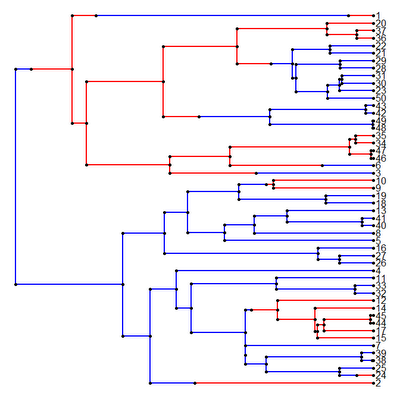> sig2<-c(1,10); names(sig2)<-cols
> # simulate rate depending on trait
> x<-sim.rates(mtree,sig2,internal=T)

And then we can plot the phenogram, including the mapped discrete character history:

> source("phenogram.R")
> phenogram(mtree,x,colors=cols)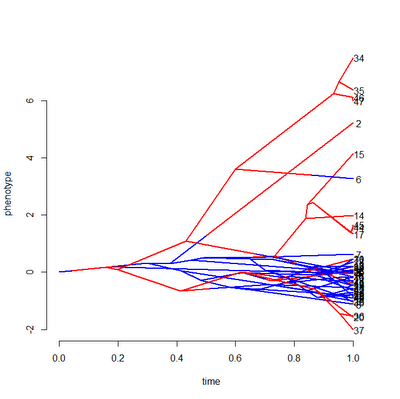The traitgram, in this case, includes the known states at internal nodes and clearly shows the difference in rate between states. If the ancestral values were not known & provided, they would be estimated using ML.

## Monday, December 19, 2011

### Faster way to compute the maximum value of Pagel's lambda

It has previously been pointed out that the maximum value of λ (such that the likelihood is still defined) for an ultrametric phylogeny can be computed as the maximum of the diagonal of vcv.phylo(tree) (or the mean, as this is a costant), divided by the maximum of the off-diagonals. In other words, we could write a function:

maxLambda<-function(tree){
C<-vcv(tree)
max(C)/max(C[lower.tri(C)])
}

Now, this function is not particularly speedy because the computation vcv.phylo(tree) is quite slow. For instance:

> tree<-drop.tip(birthdeath.tree(b=1,d=0,taxa.stop=2001),"2001")
> maxLambda<-function(tree){
+ C<-vcv(tree)
+ max(C)/max(C[lower.tri(C)])
+ }
> system.time(lambda<-maxLambda(tree))
user  system elapsed
33.65    0.20   33.93
> lambda
 1.000035

Well, I just realized we could do this much more efficiently using the function nodeHeights from the phytools package. Here, the function would look something like this:

maxLambda<-function(tree){
H<-nodeHeights(tree)
max(H[,2])/max(H[,1])
}

And, indeed, this is much faster:

> system.time(lambda<-maxLambda(tree))
user  system elapsed
0.66    0.00    0.65
> lambda
 1.000035

## Saturday, December 17, 2011

### phytools version 0.1-5 available

I just posted a new version of phytools, version 0.1-5, on my R phylogenetics page. I have also submitted this version to CRAN so, assuming it is expected, it should percolate through the mirrored repositories over the coming days. This version of the phytools library contains all the new functions and updates documented in recent posts; and in addition it includes the new function for Bayesian comparative analyses called fitBayes(). I will write a post about this function and method in the near future. Check it out, and remember, feedback (positive or negative) is always welcome.

In addition, if you have trouble with version 0.1-5 and want to try either 0.1-3 or 0.1-4, you can find them online just by typing http://faculty.umb.edu/liam.revell/phytools/older.versions/phytools_0.1-4.tar.gz (modify as appropriate) into your address bar.

### Comment on update to plotSimmap()

I just wanted to make a quick comment on my recent update to the phytools function plotSimmap() which now allows it to plot both "phylo" and "multiPhylo" SIMMAP style trees. As a preliminary, an object of class "multiPhylo" is just a list of "phylo" objects with class(...)<-"multiPhylo". To see that this is true, just make a list of "phylo" objects, set the class to "multiPhylo", and then apply any function that accepts "multiPhylo" objects to this list.

For instance, if we want to simulate 100 pure-birth trees we might want to use the geiger function birthdeath.tree. The only problem is it only simulates one tree at a time. To generate a set of (say) 100 pure-birth trees with 100 taxa, we can just do the following:

> require(geiger)
> trees<-replicate(100,birthdeath.tree(b=1,d=0,taxa.stop=100),simplify=F)
> "multiPhylo"->class(trees)
> plot.multiPhylo(trees) # we can also use the generic plot(...) here

Now, the way that plot.multiPhylo is simply by looping over each element in the "multiPhylo" object and calling the function plot.phylo(), after first setting the graphical parameter par(ask=TRUE) which will force R to ask the user for input (click or ENTER) before drawing each new tree. We can easily replicate this in plotSimmap() as follows:

plotSimmap<-function(tree,...){
if(class(tree)=="multiPhylo"){
for(i in 1:length(tree)) plotSimmap(tree[[i]],...)
} else { ...

If we do this, it works great! So for instance (after getting the new version of phytools here):

> require(geiger); require (phytools)
> tree<-rescaleTree(birthdeath.tree(b=1,d=0,taxa.stop=100),1)
> mtree<-sim.history(tree,Q=matrix(c(-1,1,1,-1),2,2))
> mtrees<-make.simmap(tree,mtree\$states,nsim=10)
> plotSimmap(mtrees)
Waiting to confirm page change...

Very cool.

## Friday, December 16, 2011

### phytools article published "Early View"Thursday December 15 was a banner day for the phytools package as in addition to my phytools phyloseminar, and unbeknownst to me until know, Methods in Ecology and Evolution has just published the phytools manuscript "Early View" (i.e., corrected proofs in advance of publication). Check it out (!); and please don't forget to cite this article when you use the phytools package in published research.

### phytools phyloseminar available online

Readers of this blog won't learn anything new from this talk (or at least my component thereof), but my "phyloseminar" with Klaus Schliep about R in phylogenetics, focusing specifically on phytools and phangorn, is now available as a recording online: phyloseminar recorded talks.

## Thursday, December 15, 2011

### New & updated phytools functions

In preparing for my recent phyloseminar, I created one new function and modified a few different existing functions. These have been posted online. [There is also a new package version that I haven't posted yet, but if you would like to try it out, you can download the source here. It contains these updates, and one other goodie in the new function fitBayes(), which I will talk about in a later post.]

The new function is the simple plotting function phenogram(), which is a lot like traitgram() in "picante", but can also take the internal node states or estimates, as well as the terminal states. The idea is really simple: we just project the phylogeny into a space defined by phenotype (in phenogram() this is the ordinate) and time (on the abscissa).

So for instance, if we try the following:

> source("phenogram.R")
> tree<-drop.tip(birthdeath.tree(b=1,d=0,taxa.stop=21),"21")
> x<-fastBM(tree)
> phenogram(tree,x,ftype="off")

we might get something that looks like this (I have turned off the tip labels here since they are inconsequential in this example):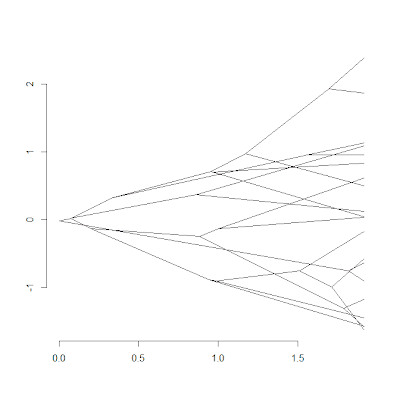This is more or less the same as you'd get with traitgram in the picante package.

The interesting thing comes when we simulate under a more complex model, say BM with a trend, and then plot the phenogram containing the true tip states and ancestral character values. We can do this, say, as follows:

> x<-fastBM(tree,mu=1,internal=T)
> phenogram(tree,x,ftype="off")1) plotSimmap() can now handle "multiPhylo" objects, in which case you just advance through the trees by hitting ENTER or clicking on the plotting window.

2) sim.rates() can now return the states for internal nodes as well as the tips of the tree.

3) brownieREML() now has a small update intended to correct the error reported here. (This fix may have to be refined further.)

As noted above, you can also a new test release of phytools containing these updates here and then install phytools from source.

## Wednesday, December 14, 2011

### Don't forget: phyloseminar tomorrow at 2PM ESTDon't forget to consider attending the phyloseminar that I am giving in collaboration with Klaus Schliep tomorrow at 2PM EST. I will be talking about the capabilities of the phytools package, and I also plan to do some simple software demonstrations. I would like to take suggestions on what functionality of phytools to demonstrate - of course, that requires that some users of the package attend the seminar! (Of course, I could always feed some questions to my grad student just in case.) Klaus will be talking about the incredible phangorn library for phylogeny inference (which I now see has a new release).

### Error message from brownie.lite and brownieREML

A user recently reported receiving the following error messages from brownieREML:

> res<-brownieREML(mtree,x)
Error in optim(rep(1, p) * runif(n = p), likelihood2, tree = tree, x = x, :
L-BFGS-B needs finite values of 'fn'

and from brownie.lite:

> res<-brownie.lite(mtree,x)
Error in solve.default(sig * C) :
Lapack routine dgesv: system is exactly singular

respectively.

The above error message is typical of trees with terminal branch lengths of zero length. This is because if two terminal taxa from an internal node have zero length branches, then the matrix vcv.phylo(tree) is singular (i.e., it has a determinant of zero and cannot be inverted). However, in the case of this user's dataset and tree, this was not the problem.

What I discovered was that the problem disappeared if I increased the scale of the data, say, by a factor of 100 or 1000. So, for instance:

> res<-brownie.lite(mtree,100*x)

worked fine.

This led me to suspect that the problem resulted from very low rates along some branches of the tree which resulted in a among species covariance matrix that was singular to numerical precision. In fact, this seems to have been the case as the fitted evolutionary rates are indeed extremely small along some of the branches of the phylogeny.

Multiplying the data by a scalar preserves the relative rates for different regimes as well as the difference in log-likelihood between different models for the scaled data; and the rates themselves are scaled by a factor of the constant squared. So, for instance, consider the following illustrative example:

> require(phytools); require(geiger)
> # simulate tree & data
> tree<-rescaleTree(drop.tip(birthdeath.tree(b=1,d=0, taxa.stop=101),"101"),1)
> Q<-matrix(c(-2,1,1,1,-2,1,1,1,-2),3,3)
> mtree<-sim.history(tree,Q)
> sig2<-c(1,10,100); names(sig2)<-c(1,2,3)
> x<-sim.rates(mtree,sig2)
> # fit model to data on the original scale
> res1<-brownie.lite(mtree,x)
> # fit to data scaled by a factor of 10
> res10<-brownie.lite(mtree,x*10)
> # compare relative rates
> res1\$sig2.multiple/res1\$sig2.multiple["1"]
2         3         1
9.575183 92.854992  1.000000
> res10\$sig2.multiple/res10\$sig2.multiple["1"]
2         3         1
9.574489 92.844736  1.000000
> # compare absolute rates
> res10\$sig2.multiple/res1\$sig2.multiple
2         3         1
99.99858  99.99477 100.00582
> # compare log-likelihood differences
> res1\$logL.multiple-res1\$logL1
 24.51724
> res10\$logL.multiple-res10\$logL1
 24.51724

## Tuesday, December 13, 2011

### Computing phylogenetic signal across multiple trees

A user just inquired as to whether there was an easy way to compute phylogenetic signal across multiple trees (say, a set of trees sampled from the posterior distribution or a set of equally parsimonious trees). In fact, this is quite easy.

To illustrate this, I will first simulate a set of 10 trees, and then I will generate data on one of these. This is intended to replicate our typical data analysis pipeline in which we have multiple trees but one vector of phenotypic trait values for species.

> require(phytools) # load phytools & ape
> trees<-rmtree(10,50) # 10 random trees
> x<-fastBM(trees[])

If we are reading data from file, x should be a vector with the phenotypic trait values for species in which names(x) contains the tip names in the tree.

Now, we can use the base function sapply() to get a vector of K values for each tree in our set (of course, in this example, all the trees except the 1st are random with respect to the phenotypic data in x).

> K<-sapply(trees,phylosig,x=x)
> K
 0.8565636 0.2462468 0.1695000 0.1727985 0.2410287 0.2135242
 0.2088892 0.1913755 0.2381923 0.1880249

We can also compute P-values if we want, using the same general approach:

> K<-sapply(trees,phylosig,x=x,test=T)
> K
[,1]      [,2]      [,3]   [,4]      [,5]      [,6]
K 0.8565636 0.2462468 0.1695 0.1727985 0.2410287 0.2135242
P 0.001     0.154     0.637  0.692     0.423     0.303
[,7]      [,8]      [,9]      [,10]
K 0.2088892 0.1913755 0.2381923 0.1880249
P 0.856     0.983     0.301     0.852

Here, sapply() just wraps our results into two rows. Of course, the same logic also applies to method="lambda", for instance:

> Lambda<-sapply(trees,phylosig,x=x,test=T,method="lambda")
> Lambda
[,1]         [,2]         [,3]         [,4]
lambda 0.9811238    5.470023e-05 6.856524e-05 6.894409e-05
logL   -78.65561    -105.1774    -100.7317    -100.9566
P      6.506385e-10 1            1            1
[,5]         [,6]       [,7]         [,8]
lambda 7.387218e-05 0.3517811  7.669448e-05 4.969137e-05
logL   -99.49018    -99.61256  -100.3459    -103.9276
P      1            0.01121933 1            1
[,9]         [,10]
lambda 7.314114e-05 7.700847e-05
logL   -101.0983    -103.7509
P      1            1

Good question. Thanks!

## Friday, December 9, 2011

### phytools & phangorn "phyloseminar" in 1 week!

Hi all. I just wanted to post a quick announcement that Klaus Schliep, author of the phangorn package, and myself will be giving a phyloseminar (that is, a participatory web seminar on phylogenetics: http://phyloseminar.org) one week from this Thursday, on Dec. 15th. The talk will start at 2pm Eastern, earlier or later in other time zones, and we are splitting about an hour long session including questions. I will both be talking about new methods as well as demonstrating the use of the phytools library in R. I hope some of the readers of this blog will attend!

## Thursday, December 8, 2011

### Minor fix to anc.ML()

Dave Bapst kindly pointed out that the function anc.ML() will fail if there are any zero length internal or terminal branches in the tree.

For zero length tip edges this is unavoidable because the determinant of vcvPhylo(tree) for this tree will always be zero. However, for zero length internal edges this can be avoided by first collapsing these using the ape function di2multi(). Unfortunately, anc.ML could not handle these trees because it uses pic() to estimate a starting value for the evolutionary rate, σ2!

Fortunately, I have resolved this by way of a couple of fixes. 1) The function first checks if the tree contains any zero length edges. If it does, then anc.ML will return an informative error message. 2) If the tree does contain polytomies, then pic() will be called on an arbitrarily resolved tree (using multi2di). The new version of this function is available here.

## Wednesday, December 7, 2011

### Dropping a random tip (or set of tips) from a tree

In a couple of recent posts (1,2) I have commented on Google search strings that (as the administrator of this blog) I can see have led users to my site. Today, a user ended up visiting the blog due to the following search string:

"randomly drop.tip in r"

by which I take it to mean they'd like to drop a random tip (or set of tips) from a "phylo" object in R.

Here's how to do it. To drop a single tip at random just type:

tr2<-drop.tip(tr1,tr1\$tip.label[ceiling(runif(n=1, max=length(tr1\$tip)))])

To drop a set of tips, say m=10 random tips, just type:

tr2<-drop.tip(tr1,sample(tr1\$tip.label)[1:m])

(actually, this will also work for a single tip, you just need to set m=1). Of course, if you wanted to, say, drop 30% of the taxa at random, you would just have to first compute the number of taxa to drop, m, and then apply the code above. For instance:

m<-round(0.3*length(tr1\$tip))
tr2<-drop.tip(tr1,sample(tr1\$tip.label)[1:m])

It's that easy!

### Ancestral state estimation when the rate of evolution varies throughout the tree

A user contacting me recently about performing ancestral state estimation when the rate of evolution varies on the different branches of the tree. (Actually, she contacted me about another, slightly more complex problem - but this is where I'm starting.) To see why this might be an issue, consider the following tree:and imagine that the rate of evolution is very low [say σ(blue)2=0.01] on the blue branches of the tree, and very high [say σ(red)2=1.0] on the red branches. Under this scenario, the states at the internal nodes of the tree will be much closer in value to the state at the root node than they will be (on average) to any red tip. We might like to take this into consideration in estimating ancestral character values.

To show this, let's consider the following example. First, let's simulate data on the tree by first stretching the branches of the tree by their rates of evolution, then by using fastBM(). Here I use fastBM() rather than the much easier phytools function sim.rates(), because fastBM() allows us to return the states at internal nodes. Then, I will use anc.ML() to estimate internal node states using likelihood, ignoring heterogeneity in the evolutionary rate, and compare these estimates to the "true," normally unknown, ancestral character values.

> require(phytools)
> source("anc.ML.R")
> cols<-c("blue","red"); names(cols)<-c(1,2)
> sig2<-c(0.01,1); names(sig2)<-c(1,2)
> simtree<-tree; simtree\$edge.length<-colSums(t(tree\$mapped.edge)*sig2)
> x<-fastBM(simtree,internal=T) # parts of Newick style tree not shown
> ace1<-anc.ML(tree,x[1:32])
> mse1<-mean((x[33:length(x)]-ace1\$ace)^2)
> mse1
 0.09184913
> plot(x[33:length(x)],ace1\$ace)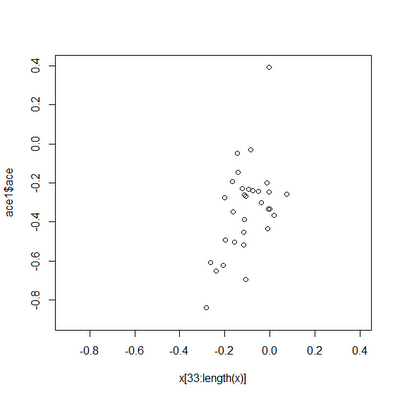Obviously, these estimates are not great, and the ancestral state estimates (on the y-axis) span a much broader range than the true values, as we might expect. [I have plotted the points on a square set of axes just to make this point clear.] Now, for comparison, let's first fit a multi-rate BM model and then try to use the fitted rates to inform our ancestral character estimation on the tree:

> fit<-brownie.lite(tree,x[1:32])
> fit
...
\$sig2.multiple
1 2
0.005546321 0.684734146
...
\$P.chisq
 2.143502e-11
> fittree<-tree
> fittree\$edge.length<- colSums(t(tree\$mapped.edge)*fit\$sig2.multiple)
> ace2<-anc.ML(fittree,x[1:32])
> mse2<-mean((x[33:length(x)]-ace2\$ace)^2)
> mse2
 0.005524579
> plot(x[33:length(x)],ace2\$ace)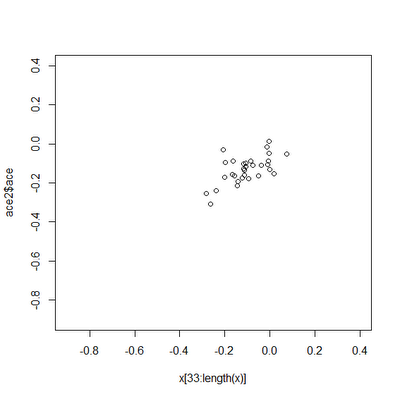Clearly, the estimates are much closer to the true values in this case.

To be perfectly transparent, this particular example is designed to be an extreme case, and for most trees & datasets, the effect will be much smaller.

## Tuesday, December 6, 2011

### Ancestral character estimation using likelihood

I just posted a new function for ancestral character estimation using likelihood called anc.ML(). (Direct link to code here.) This function is a lightweight version of ace() from the ape package, in that it only does likelihood estimation and only for continuously valued character traits. I developed this because I have been having trouble with ace(...,method="ML") in terms of accuracy. In particular, with some regularity (maybe 5% of the time on stochastic 100 taxon trees), the ML optimization in ace() seems to converge to the wrong ancestral character values.

One difficulty that I encountered when trying to evaluate the performance of anc.ML() was the discovery that the likelihoods reported by both functions are different. In particular, I realized that the likelihoods reported by ace() exclude constant terms. For a Brownian model, the constant terms for a set of ancestral states and rate for the BM process are two: –(n+m-1)log(2π)/2 (for n species and m internal nodes), and log(|C|)/2 (for cophenetic matrix C). Sure enough, this is exactly the amount by which the log-likelihoods returned by ace(...,method="ML") and anc.ML() differ.

First, an example when ace() is working:

> require(phytools) # needs phytools 0.1-2
> source("anc.ML.R") # load the source code for anc.ML
> set.seed(1)
> tree<-rtree(100)
> x<-fastBM(tree)
> res1<-ace(x,tree,method="ML")
> res1\$loglik
 -29.04148
> res2<-anc.ML(tree,x,maxit=4000)
> res2\$logLik
 -116.1555
> plot(res1\$ace,res2\$ace)
> res1\$loglik-(length(tree\$tip)+tree\$Nnode-1)*log(2*pi)/2-as.numeric(determinant(vcvPhylo(tree),logarithm=T)\$modulus)/2
 -116.1555This just shows that ace(...,method="ML") and anc.ML() produce the same estimates (to a fairly high degree of precision); and that the likelihoods differ by a constant for a given tree. That is, of course, when ace() behaves. Sometimes it doesn't. Try this example:

> set.seed(90)
> tree<-rtree(100)
> x<-fastBM(tree)
> res1<-ace(x,tree,method="ML")
> res1\$loglik-(length(tree\$tip)+tree\$Nnode-1)*log(2*pi)/2-as.numeric(determinant(vcvPhylo(tree),logarith=T)\$modulus)/2
 -92.44219
> res2<-anc.ML(tree,x,maxit=4000)
> res2\$logLik
 -84.40321
> plot(res1\$ace,res2\$ace)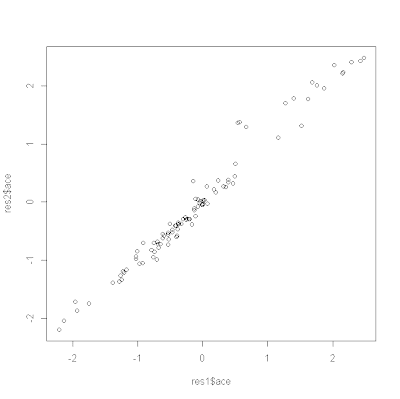Obviously, something is amiss in this example. I was working on this to show how to do ancestral state estimation for a continuous trait when rates of evolution differ in different parts of the tree, and I will try to iron out any bugs in anc.ML as I work on this.

## Saturday, December 3, 2011

### Dropping the same tip from many trees in a "multiPhylo" object

Following the same theme as an earlier post, I thought I would take a closer look a Google search strings that have led readers to my site. A recent search string that has multiple hits is the following: "drop.tip multiphylo", by which I presume they mean to ask how one drops the same tip (or set of tips) from a set of phylogenies in an object of class "multiPhylo". It turns out that we can do this very easily, in fact.

First, let's get a set of trees as an object of the class "multiPhylo". [In most cases, of course, these would probably be trees we have read in from file (say, as a posterior sample of trees in a Bayesian analysis). In this case, we will just use the ape function rmtree().]

> require(ape)
> trees<-rmtree(N=10,n=20)
> trees
10 phylogenetic trees
> require(phytools)
> plotTree(trees[]) # for instanceThis gives a set of 10 trees, each containing 20 taxa, with the tip labels "t1" to "t20".

Now, a "multiPhylo" object is really just a list of trees, so to apply the ape function drop.tip to all the trees in the list, we can just use the function lapply. Let's drop, say "t13" (for luck) from all the trees in the list:

> ntrees<-lapply(trees,drop.tip,tip="t13")
> class(trees)<-"multiPhylo"
> plotTree(ntrees[]) # for comparison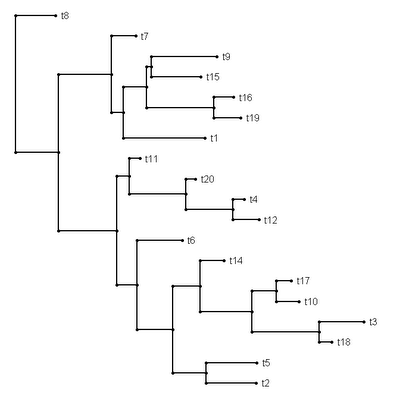and taxon "t13" is gone, just as we'd hope. (The same is true, of course, of all the trees in our set.)

Naturally, the same technique works for a set of tips, for instance:

> ntrees<-lapply(trees,drop.tip,tip=c("t11","t12","t13"))
> class(ntrees)<-"multiPhylo"

We can even combine this with the trick, described in an earlier post that allows us to drop all but the set of taxa we have in a list. In this case, we will make the special function keep.tip. For instance if we wanted to keep only "t1" through "t10" in each of our trees above, we could just do the following:

> species<-paste("t",1:10,sep="")
> species
 "t1" "t2" "t3" "t4" "t5" "t6" "t7" "t8" "t9" "t10"
> keep.tip<-function(tree,tip) drop.tip(tree,setdiff(tree\$tip.label,tip))
> ntrees<-lapply(trees,keep.tip,tip=species)
> "multiPhylo"->class(ntrees)
> plotTree(ntrees[])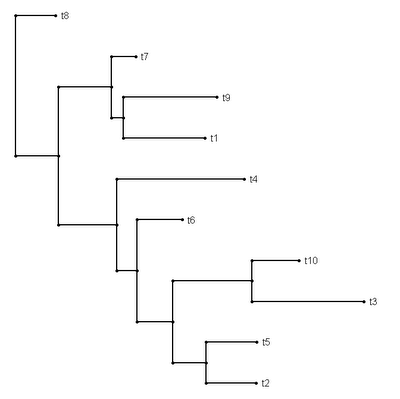[Thanks to Dan Rabosky for the setdiff() suggestion.] Cool.

## Thursday, December 1, 2011

### Testing for phylogenetic signal (K) different than 1.0

I can see as administrator of this blog some of the google search terms that lead users to the site. Today, someone ending up on the phytools blog with the following search: "phylogenetic signal k significantly different than one."

Testing for whether K (Blomberg et al. 2003) is significantly different from 1.0 is not explicitly implemented in my function phylosig(), but it is straightforward to imagine how one would go about doing it with simulation.

First, let's simulate data with strong phylogenetic signal (expected K of 1.0):

> require(phytools)
> tree<-rtree(100)
> x<-fastBM(tree)
> K<-phylosig(tree,x)
> K
 1.103667

Now, let's get a null distribution for K=1.0 via simulation on our phylogeny:

> nullK<-apply(fastBM(tree,n=1000,sig2=mean(pic(x,tree)^2)),2, phylosig,tree=tree)

Finally, let's count the number of simulated K values that are more extreme than our observed value for K. I would suggest that we do this by counting the number of times that the absolute value of the logarithm of our observed value for K is no smaller than the absolute values of our simulated values for K. This just makes K=1.5 & K=0.6667 equivalent departures from BM. I.e.,

> P<-mean(abs(log(c(K,nullK)))>=abs(log(K)))
> P
 0.8011988

Ok, now let's contrast this with data generated with no signal.

> x<-rnorm(n=length(tree\$tip)); names(x)<-tree\$tip.label
> K<-phylosig(tree,x)
> K
 0.1998674
> nullK<-apply(fastBM(tree,n=1000,sig2=mean(pic(x,tree)^2)),2, phylosig,tree=tree)
> P<-mean(abs(log(c(K,nullK)))>abs(log(K)))
> P
 0.000999001

Makes sense.

### Percent variance from phyl.pca()

A user just emailed me to find out how to get the "percent variance explained" by each eigenvector from phytools' phylogenetic PCA (phyl.pca function. This is easy, just do the following:

> require(phytools)
> res<-phyl.pca(tree,X)
> diag(res\$Eval)/sum(res\$Eval)*100
PC1      PC2      PC3      PC4      PC5
26.83767 23.78144 20.18687 15.85040 13.34361

Cool.

## Sunday, November 27, 2011

### Simulating an irreversible discrete trait using sim.history()

A user asked how one should go about simulating a character that evolves from state "0" (say) to state "1", but not the reverse. This corresponds to the following transition matrix:

> Q
0 1
0 -1 0
1  1 0

in which we can substitute any instantaneous transition rate for the 1.0 and -1.0. Unfortunately, for various reasons sim.history doesn't work for transition rates of exactly zero - however it works fine if we substitute a value slightly greater than zero. To see this work, try the following example code that uses "geiger" and "phytools":

require(phytools)
require(geiger)
tree<-drop.tip(birthdeath.tree(b=1,d=0,taxa.stop=101),"101")
tree<-rescaleTree(tree,1)
Q<-matrix(c(-1,1,1e-10,-1e-10),2,2)
rownames(Q)<-colnames(Q)<-c("0","1")
mtree<-sim.history(tree,Q,"0")
cols<-c("blue","red"); names(cols)<-c(0,1)
plotSimmap(mtree,cols,ftype="off")

which should produce something that looks like this:Don't forget, of course, that the tip states are stored in mtree\$states, i.e.:

> mtree\$states
53  98  99  24  25  26  18  13  30  31  56  80
"0" "0" "0" "0" "0" "0" "0" "0" "1" "1" "1" "1"
81  77  ...
"1" "1"  ...

## Saturday, November 26, 2011

### phytools version 0.1-2

I just posted a new version of phytools, version 0.1-2, to my webpage, here. I have also submitted this version to CRAN (but it will probably take a day or two to be accepted, and then a few days more to percolate through all the mirror repositories). In the meantime, it can be installed in Windows by downloading the file phytools_0.1-2.zip and then typing:

> install.packages("phytools_0.1-2.zip",repos=NULL)

at the prompt. (For instructions on how to install on Mac/Linux/Unix from source, please see my website.)

New features in this version of phytools include:

1) A new function, anc.Bayes(), for Bayesian ancestral state estimation.

2) A new function, vcvPhylo(), to compute the so-called phylogenetic variance covariance matrix including ancestral nodes. I posted about this function here.

Check it out!

## Friday, November 25, 2011

### Major speed up of anc.Bayes()

I just realized how to score a major speed-up in computation time from my new Bayesian ancestral character estimation function for continuous traits (anc.Bayes). Last night I documented what I thought at the time was a significant speed improvement. By pulling inversion of the phylogenetic VCV matrix out of the likelihood function (meaning that we only have to do this once instead of every generation of the MCMC), I was able to cut computation time in half (from about 60s on a 100 taxon tree to about 30s). What I didn't realize at the time was that I could also pull out calculation of the determinant of the matrix. The determinant we want is log(|σ2C|); the way we avoid recalculating this every generation of the MCMC (in which σ2 can potentially differ) is by taking advantage of the equality:

log(|σ2C|) = nlog(σ2) + log(|C|)

I actually described this in an earlier paper with Luke Harmon (Revell & Harmon 2008, p. 318), but did not think to apply it to this problem until now. [Note that there is actually a small error in equation (5) of our article: n·r·log(k) should be n·r·log(k)/2.]

This version runs much faster: now about 4s for the same dataset as before. Unbelievable! Anyway, I have posted the new version on my website here.

This version is so much faster that I can easily run it for a million generations on the 100 species tree from before. If I plot the estimates from the Bayesian MCMC run with an uninformative prior against the values from ML estimation using ace() in the ape package, we can see that they are even more tightly correlated than before:

> source("anc.Bayes.R")
> source("vcvPhylo.R")
> X<-anc.Bayes(tree,x,ngen=1000000)
> plot(ace(x,tree)\$ace,colMeans(X[21:10001,])[2+1:tree\$Nnode])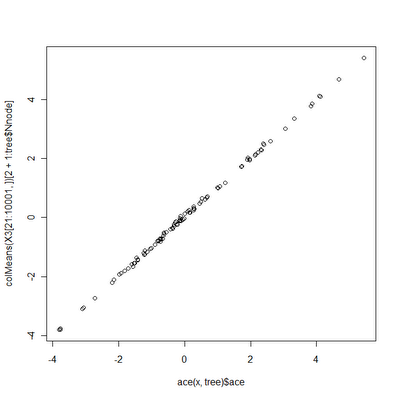(compare to here). The above code took about 6 minutes to run, whereas the first version of anc.Bayes would've needed about 100 minutes for the same calculations.

I haven't mentioned MCMC diagnostics, priors, or the utility of Bayesian inference at all yet. Hopefully, these will be addressed in future posts.

### Improvement to anc.Bayes()

I have already identified a major area in which I can easily improve the computation time of anc.Bayes(), my new function for Bayesian MCMC ancestral state estimation. Specifically, after having thought myself quite clever for using dmnorm() in the mnormt library (see post here), I realized that this was actually extremely computationally inefficient - especially for large matrices. This is because by using dmnorm() I'm essentially forced R to invert our VCV matrix (which is of order number of tips + number of nodes) every generation of the MCMC. This is totally unnecessary and I can easily create a custom function to compute the MVN probability density in which I avoid inverting the phylogenetic VCV matrix on each call. This function is below (it still requires that we invert C once), and I will post a new version of anc.Bayes() tomorrow.

likelihood<-function(C,invC,x,sig2,a,y){
logLik<--(c(x,y)-a)%*%invC%*%(c(x,y)-a)/(2*sig2) -nrow(invC)*log(2*pi)/2 -determinant(sig2*C,log=T)\$modulus/2
return(logLik)
}

## Thursday, November 24, 2011

### Bayesian ancestral character estimation

I've just been working on a new function for Bayesian ancestral character estimation (anc.Bayes). I'm still working out some of the bugs, but I have nonetheless posted a preliminary version online, here. (It also uses vcvPhylo(), which can be downloaded here).

1) If we take advantage of the multivariate normal density function dmnorm in the mnormt package, and my function vcvPhylo(), computing the likelihood (which we will use to calculate the posterior odds ratio) can be done in just a few lines of code:

C<-vcvPhylo(tree)
# function returns the log-likelihood
likelihood<-function(C,x,sig2,a,y){
logLik<-dmnorm(x=c(x,y),mean=rep(a,nrow(C)),varcov=sig2*C, log=TRUE)
return(logLik)
}

in which x is the data for species, y are the ancestral character values, a is the state at the root node, and sig2 is the evolutionary rate.

2. All the control parameters of the Bayesian MCMC (starting values, proposal distributions, and prior probabilities) are accessible to the user - but I have also tried to give the function the capacity to give reasonable values for these things if they are not provided by the user.

Although I'll warn again that this I am still working on this, it is not too soon to try it out. Let's simulate, run the MCMC, and then compare the results to ML estimates obtained using ace() in the ape package:

> require(phytools)
> source("vcvPhylo/vcvPhylo.R")
> source("anc.Bayes/anc.Bayes.R")
> set.seed(100)
> tree<-rtree(100)
> x<-fastBM(tree,sig2=2)
> result<-anc.Bayes(tree,x,ngen=100000)
Control parameters (set by user or default):
List of 7
\$ sig2   : num 2.03
\$ a      : num [1, 1] 0.00737
\$ y      : num [1:98] 0.00737 0.00737 0.00737 0.00737 0.00737 ...
\$ pr.mean: num [1:100] 1000 0 0 0 0 0 0 0 0 0 ...
\$ pr.var : num [1:100] 1e+06 1e+03 1e+03 1e+03 1e+03 1e+03 1e+03 1e+03 1e+03 1e+03 ...
\$ prop   : num [1:100] 0.142 0.142 0.142 0.142 0.142 ...
\$ sample : num 100
Starting MCMC...
Done MCMC.
> # plot the result against the MLEs
> plot(ace(x,tree)\$ace,colMeans(result[21:1001,])[2+1:tree\$Nnode])

Here, we get the following result: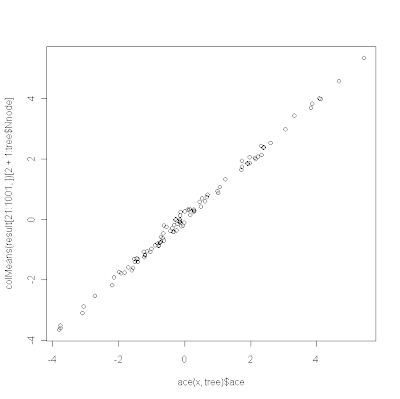Obviously, I will post more on this as I improve it and I will also try to provide more guidance on MCMC control and priors.

## Wednesday, November 23, 2011

### Faster vcvPhylo() with ancestral nodes

In my last post I put up a simple version vcv.phylo() (which returns a matrix containing the height above the root of each pair of species in the tree, sometimes called the phylogenetic variance-covariance matrix), but that included ancestral nodes. I pulled this out of an old function of mine, anc.trend(), so I figured I could do better - and after some tinkering I came up with a much improved version, as follows:

vcvPhylo2<-function(tree,anc.nodes=T){
n<-length(tree\$tip.label)
h<-nodeHeights(tree)[order(tree\$edge[,2]),2]
h<-c(h[1:n],0,h[(n+1):length(h)])
M<-mrca(tree,full=anc.nodes)[c(1:n,anc.nodes*(n+2:tree\$Nnode)),c(1:n,anc.nodes*(n+2:tree\$Nnode))]
C<-matrix(h[M],nrow(M),ncol(M))
if(anc.nodes) rownames(C)<-colnames(C)<-c(tree\$tip.label,n+2:tree\$Nnode)
else rownames(C)<-colnames(C)<-tree\$tip.label
return(C)
}

A couple of tricks in here:

1) Rather than calling dist.nodes(), this function uses the phytools function nodeHeights, which returns a matrix of the dimensions of tree\$edge, containing the height above the root of every node, and is quite fast.

2) The function then uses mrca(...,full=T) in the ape package, which returns the MRCA of every pair of species and nodes. This essentially serves as an index to construct our VCV matrix from the node heights!

All of this is done with the following lines of code:

# this gets a vector of height by node number
h<-nodeHeights(tree)[order(tree\$edge[,2]),2]
# this inserts a height of zero for the root node (node n+1 for n species)
h<-c(h[1:n],0,h[(n+1):length(h)])
# this is our index matrix
M<-mrca(tree,full=T)
# this is our VCV matrix
C<-matrix(h[M],nrow(M),ncol(M))

Let's compare computation time to vcv.phylo and our earlier version (vcvPhylo1):

> tree<-rtree(500)
> system.time(X<-vcv.phylo(tree)) # only tips
user  system elapsed
2.20    0.00    2.22
> system.time(Y<-vcvPhylo1(tree)) # tips & nodes, old version
user  system elapsed
7.68    0.03    7.73
> system.time(Z<-vcvPhylo2(tree)) # tips & nodes, new version
user  system elapsed
3.49    0.05    3.54
> all(Y==Z)
 TRUE
> all(X==Z[c(1:500),c(1:500)])
 TRUE

Cool! BTW - Happy Thanksgiving to all the blog readers.

## Monday, November 21, 2011

### vcv.phylo() with ancestral nodes

An R-sig-phylo user just posted a request for a function to compute the so-called phylogenetic variance-covariance matrix. That is, the N×N matrix (for N species) containing in position i,j the height above the root of the MRCA of species i and j (as done in the ape function vcv.phylo) - but including ancestral nodes. As it turns out, I have a code fragment to do this in my function anc.trend() (link here). I pasted the fragment into a function vcvPhylo() as follows:

vcvPhylo<-function(phy,anc.nodes=T){
if(anc.nodes){
D<-dist.nodes(phy)
ntips<-length(phy\$tip.label)
Cii<-D[ntips+1,]
C<-D; C[,]<-0
for(i in 1:nrow(D)) for(j in 1:ncol(D))
C[i,j]<-(Cii[i]+Cii[j]-D[i,j])/2
dimnames(C)[][1:length(phy\$tip)]<-dimnames(C)[][1:length(phy\$tip)]<-phy\$tip.label
C<-C[c(1:ntips,(ntips+2):nrow(C)),c(1:ntips,(ntips+2):ncol(C))]
return(C)
} else return(vcv.phylo(phy))
}

and it seems to do the trick.

## Thursday, November 17, 2011

### phytools version 0.1-1

I just released the latest version of phytools to my website, here. The new features and functions of this version include:

1) A new function, make.era.map(), which allows the user to map temporal eras on the tree. More details in a prior post.

2) A user requested update to phyl.RMA() allowing for a fixed value of the λ parameter. More on this here.

3) A new function, brownieREML, which does much faster REML model fitting of the multiple rate Brownian evolution model of O'Meara et al. (2006). More on this here.

4) A couple of updates to phylosig() that allow the incorporation of sampling error into estimates of phylogenetic signal using K & λ. The method for including sampling error is based on Ives et al. (2007).

I also added a CITATION file. The CITATION file tells R how to respond to the function call:

> citation("phytools").

My CITATION file looks something like the following:

citHeader("To cite phytools in publication use:")
citEntry(
entry="Manual",
title="phytools: An R package for phylogenetic comparative
biology (and other things).",
author="Liam J. Revell",
year=2012,
url="http://cran.r-project.org/web/packages/phytools/",
textVersion=paste("Revell, L. J. (In press)","phytools: An R package for phylogenetic comparative biology (and other things)","Methods Ecol. Evol.")
)
citFooter("As phytools is continually evolving, you may want to cite its version number. Find it with 'help(package=phytools)'.")

and calls to citation("phytools") will now return the following:

> citation("phytools")

To cite phytools in publication use:

Revell, L. J. (In press) phytools: An R package for phylogenetic
comparative biology (and other things) Methods Ecol. Evol.

A BibTeX entry for LaTeX users is

@Manual{,
title = {phytools: An R package for phylogenetic comparative
biology (and other things).},
author = {Liam J. Revell},
year = {2012},
url = {http://cran.r-project.org/web/packages/phytools/},
}

As phytools is continually evolving, you may want to cite its version number. Find it with 'help(package=phytools)'.

Cool.

## Wednesday, November 16, 2011

### Including "measurement error" in the estimation of λ

I just posted a new version of the function phylosig() which now allows the user to incorporate measurement error in the estimation of Pagel's λ. Pagel's λ is usually estimated using maximum likelihood, so it was straightforward to condition the estimation of λ & σ2 (the rate of evolution) on an observed set of standard errors. The code for this new version of the function is here. Unfortunately, as I have added capabilities to this function, I have done so in a haphazard fashion, which has resulted in code for this function that has become a little bit more expansive than necessary (compare, for instance, to the code for my new REML version of brownie.lite, here). At some point I will try and clean this up.

To add measurement error to the estimation of λ is not too difficult. Basically, under the λ model we expect that the multivariate distribution of phenotypic values among species will be given by σ2Cλ; where Cλ is an n×n matrix (for n species) containing, in the diagonal, the height of each species above the root; and, in each off-diagonal element Cλ(i,j), the height above the root node of the MRCA of species i and species j multiplied by the coefficient λ. In other words: x ~ MVN(σ2Cλ). Given a set of values for x and a tree, we can estimate λ using likelihood.

To incorporate measurement error (that is, estimation error for the species means for the trait of interest), we just have x ~ MVN(σ2Cλ + E), where E is a matrix containing the error variance and covariances among species. If we assume that estimation error is uncorrelated among species, then E is just a diagonal matrix containing the square of the estimation error for each species. (The assumption that measurement error is independent among species is reasonably safe - but it might be false if, for instance, samples were measured by different investigators or in different years; e.g., see Ives et al. 2007).

The way to run phylosig() with measurement error is simply by supplying the additional vector, se, containing the standard errors for each species. names(se) should contain the species names (corresponding to tree\$tip.label, although they need not be in the same order).

To see how the function works, let's try the following example:

> require(phytools); require(geiger)
> set.seed(2)
> gen.lambda<-0.7
> tree<-drop.tip(birthdeath.tree(b=1,d=0,taxa.stop=501),"501")
> se<-sqrt(rchisq(n=500,df=2)); names(se)<-tree\$tip.label
> x<-fastBM(lambdaTree(tree,gen.lambda),sig2=1)
> e<-rnorm(n=500,sd=se)
> xe<-x+e
> phylosig(tree,x,method="lambda")
\$lambda
 0.7141959
\$logL
 -1018.6
> phylosig(tree,xe,method="lambda")
\$lambda
 0.5431885
\$logL
 -1153.855
> phylosig(tree,xe,method="lambda",se=se)
\$lambda
 0.7274975
\$sig2
 1.161162
\$logL
 -1135.698

1) The decrease in estimated phylogenetic signal when measurement error is ignored is exactly what we expect for the λ model. This is because measurement error and the λ model have exactly the same effect on the distribution of variation among species (that is, both add extra variance to the tips).

2) The likelihoods for the first result cannot be compared to the likelihoods for the second or third result because it has been obtained for different data (in this case, the hypothetical data for species means without error - the data we never actually have in empirical studies). The likelihoods for results 2 & 3 can be compared because they are obtained for the same data. In this case, the likelihood improved dramatically for the model with measurement error. This suggests that the tree & measurement errors are much better at explaining the observed data than is the tree alone, under the λ model.

## Tuesday, November 15, 2011

### REML version of brownie.lite

I just posted a new, simplified REML version of brownie.lite(). brownie.lite() is based on the method of O'Meara et al. (2006) in which we fit different evolutionary rates to different, pre-defined parts of a phylogenetic tree with branch lengths.

The REML version works by using a likelihood function for the phylogenetically independent contrasts rather than for the original data. We optimize the multi-rate model, in this case, by optimization the (restricted) likelihood of different rescaling of the different branch lengths of the tree. The problem with maximizing the likelihood for the original data is that it involves constructing and inverting matrices of dimension n×n for n species. By maximizing a likelihood function for the contrasts, we can avoid this very computationally intensive operation. In addition, REML (unlike ML) produces unbiased parameter estimates. ML estimates are biased downward by a factor of n/(n-1).

To see the improvement in computation time that is provided by REML in this case, download the source file (here), and try out the following exercise. (Note that on a tree with 1000 terminal species, as in this example, be forewarned that brownie.lite() will take several minutes to run.)

> require(geiger); require(phytools)
> set.seed(10) # for repeatability
> tree<-drop.tip(birthdeath.tree(b=1,d=0,taxa.stop=1001),"1001")
> tree<-rescaleTree(tree,1)
> tree<-sim.history(tree,matrix(c(-1,1,1,-1),2,2))
> x<-sim.rates(tree,c(1,10))
names absent from sig2: assuming same order as \$mapped.edge
> system.time(result1<-brownie.lite(tree,x))
user  system elapsed
353.53    3.39  357.70
> source("brownieREML.R")
> system.time(result2<-brownieREML(tree,x))
user  system elapsed
0.28    0.00    0.28
> result1
\$sig2.single
 6.477488
...
\$logL1
 -1348.224
...
\$sig2.multiple
1        2
1.010363 9.819315
...
\$logL.multiple
 -1160.006
...
> result2
\$sig2.single
 6.483972
\$logL1
 -1347.577
\$sig2.multiple
1        2
1.012534 9.824353
\$logL2
 -1159.737
\$convergence
 TRUE

I anticipate that brownieREML() will be added to future versions of the "phytools" library.

## Friday, November 11, 2011

### phytools manuscript accepted

Quick post - the manuscript describing "phytools" was recently accepted at Methods in Ecology & Evolution. See blog post here.

### New version of phyl.RMA() with fixed lambda

I just posted a new version of my R function for phylogenetic reduced major axis regression (RMA), called phyl.RMA(). Since the RMA slope is merely computed as the variance ratio, this function just computes phylogenetic variances and then takes their ratio. The function also (for method="lambda") first performs joint estimation of Pagel's λ (e.g., see Freckleton et al. 2002). Direct link to the code is here.

The new feature of this version of the function (as requested by a user) is merely that the value of λ can be fixed by the user rather than estimated. This is done by calling phyl.RMA(...,fixed=TRUE,lambda=XX). So, for instance, try the following:

> require(geiger)
> require(phytools)
> source("phyl.RMA.R")
> tree<-drop.tip(birthdeath.tree(b=1,d=0,taxa.stop=201),"201")
> X<-fastBM(lambdaTree(tree,0.7),nsim=2)
> phyl.RMA(X[,1],X[,2],tree,method="lambda")
\$RMA.beta
 -0.0866432 1.0590274
\$V
x y
x 0.97406967 -0.03095092
y -0.03095092 1.09245711
\$lambda
 0.734092
\$logL
 -770.6879
\$resid
[,1]
21 -5.863653843
101 -5.841695560
...

If we wanted to know, say, if the estimated value of λ (here ~0.73) was significantly different than 0.0 (or any other value), we can fix λ at 0.0 and recalculate:

> phyl.RMA(X[,1],X[,2],tree,method="lambda",fixed=T,lambda=0)
\$RMA.beta
 -0.7000868 0.9720490

\$V
x y
x 0.75527418 -0.09025328
y -0.09025328 0.71364296

\$lambda
 0

\$logL
 -845.7699

\$resid
[,1]
21 -5.01116336
101 -4.87370275
...

Cool!

Unfortunately, it has just been reported to me that we have had an escaped anole from the common garden Anolis carolinensis rearing experiment that is presently taking place in my lab. Time to go lizard hunting!